# How to Calculate and Solve for Time Required to Irrigate | Irrigation Water Requirement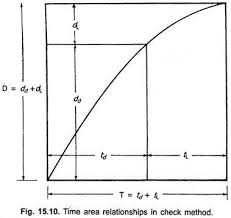The image above represents time required to irrigate.

To compute for time required to irrigate, three essential parameters are needed and these parameters are Area of the Land (A), Depth of the Water to be Applied (dwand Discharge of the Stream (q).

The formula for calculating time required to irrigate:

t = A . dw / q

Where:

t = Time Required to Irrigate
A = Area of the Land
dw = Depth of the Water to be Applied
q = Discharge of the Stream

Let’s Solve an example;
Find the time required to irrigate when the area of the land is 14, the depth of the water to be applied is 20 and the discharge of the stream is 10.

This implies that;

A = Area of the Land = 14
dw = Depth of the Water to be Applied = 20
q = Discharge of the Stream = 10

t = A . dw / q
t = 14 . 20 / 10
t = 280 / 10
t = 28

Therefore, the time required to irrigate is 28.

Calculating for the Area of the Land when the Time Required to Irrigate, the Depth of the Water to be Applied and the Discharge of the Stream is Given.

A = t x q / dw

Where;

A = Area of the Land
t = Time Required to Irrigate
dw = Depth of the Water to be Applied
q = Discharge of the Stream

Let’s Solve an example;
Find the area of the Land when the time required to irrigate is 20, the depth of the water to be applied is 12 and the discharge of the stream is 3.

This implies that;

t = Time Required to Irrigate = 20
dw = Depth of the Water to be Applied = 12
q = Discharge of the Stream = 3

A = t x q / dw
A = 20 x 3 / 12
A = 60 / 12
A = 5

Therefore, the area of the land is 5.

Calculating for the Depth of the Water to be Applied when the Time Required to Irrigate, the Area of the Land and the Discharge of the Stream is Given.

dw = t x q / A

Where;

dw = Depth of the Water to be Applied
t = Time Required to Irrigate
A = Area of the Land
q = Discharge of the Stream

Let’s Solve an example;
Find the depth of the water to be applied when the time required to irrigate is 15, the area of the land is 14 and the discharge of the stream is 12.

This implies that;

t = Time Required to Irrigate = 15
A = Area of the Land = 14
q = Discharge of the Stream = 12

dw = t x q / A
dw = 15 x 12 / 14
dw = 180 / 14
d = 12.85

Therefore, the depth of the water to be applied is 12.85.

Calculating for the Discharge of the Stream when the Time Required to Irrigate, the Area of the Land and the Depth of the Water to be Applied is Given.

q = A . dw / t

Where;

q = Discharge of the Stream
t = Time Required to Irrigate
A = Area of the Land
dw = Depth of the Water to be Applied

Let’s Solve an example;
Find the discharge of the stream when the time required to irrigate is 20, the area of the land is 10 and the depth of the water to be applied is 8.

This implies that;

t = Time Required to Irrigate = 20
A = Area of the Land = 10
dw = Depth of the Water to be Applied = 8

q = A . dw / t
q = 10 . 8 / 20
q = 80 / 20
q = 4

Therefore, the discharge of the stream is 4.

Nickzom Calculator – The Calculator Encyclopedia is capable of calculating the time require to irrigate.

To get the answer and workings of the time required to irrigate using the Nickzom Calculator – The Calculator Encyclopedia. First, you need to obtain the app.

You can get this app via any of these means:

To get access to the professional version via web, you need to register and subscribe for NGN 2,000 per annum to have utter access to all functionalities.
You can also try the demo version via https://www.nickzom.org/calculator

Android (Paid) – https://play.google.com/store/apps/details?id=org.nickzom.nickzomcalculator
Android (Free) – https://play.google.com/store/apps/details?id=com.nickzom.nickzomcalculator
Apple (Paid) – https://itunes.apple.com/us/app/nickzom-calculator/id1331162702?mt=8
Once, you have obtained the calculator encyclopedia app, proceed to the Calculator Map, then click on Agricultural under Engineering.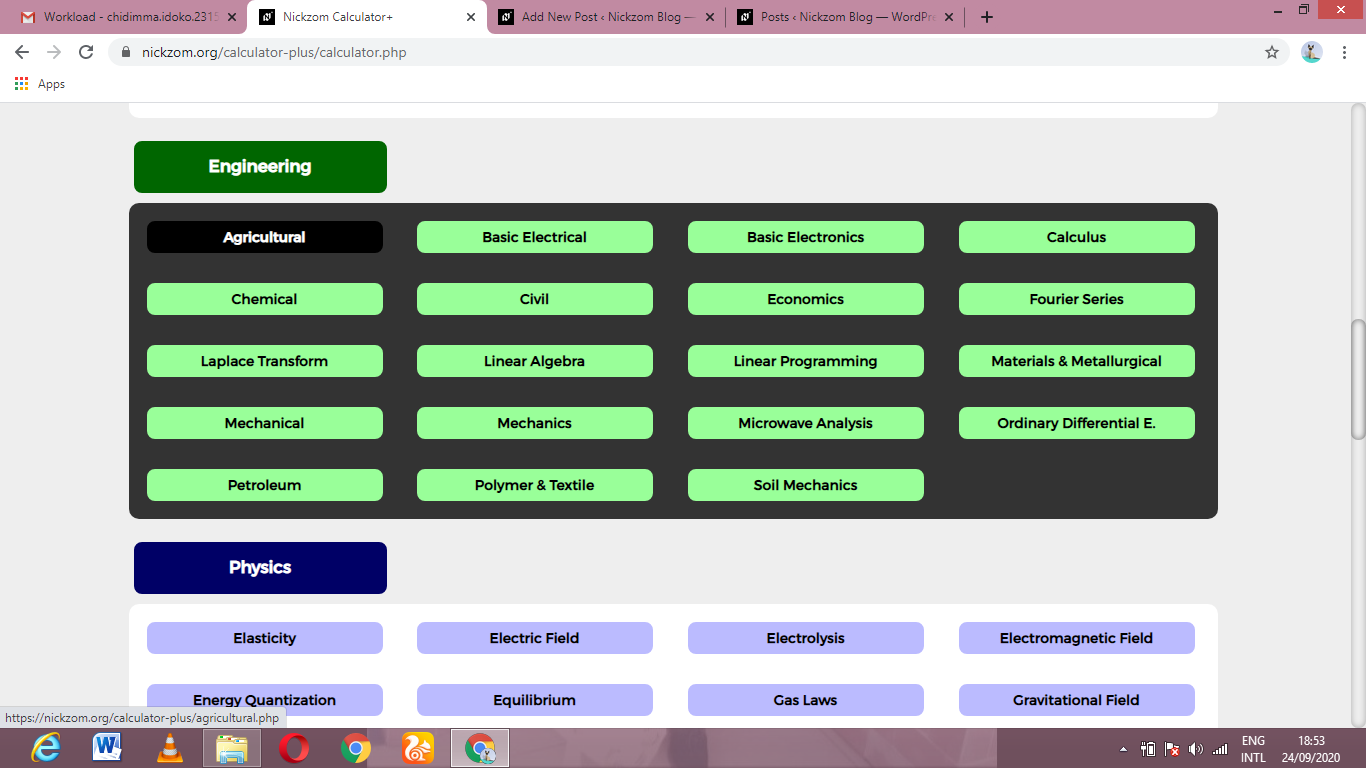Now, Click on Irrigation Water Requirement under Agricultural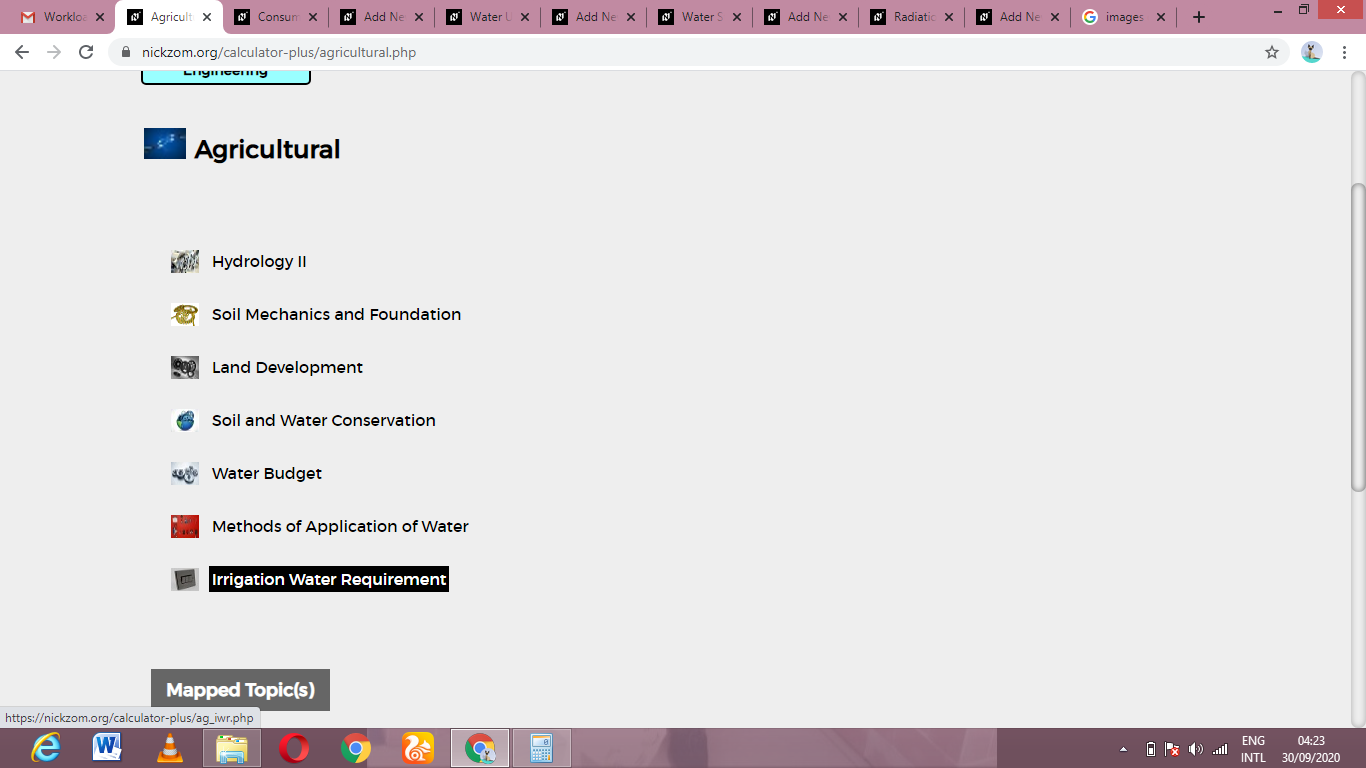Now, Click on Time Required to Irrigate under Irrigation Water RequirementThe screenshot below displays the page or activity to enter your values, to get the answer for the time required to irrigate according to the respective parameters which is the Area of the Land (A), Depth of the Water to be Applied (dwand Discharge of the Stream (q).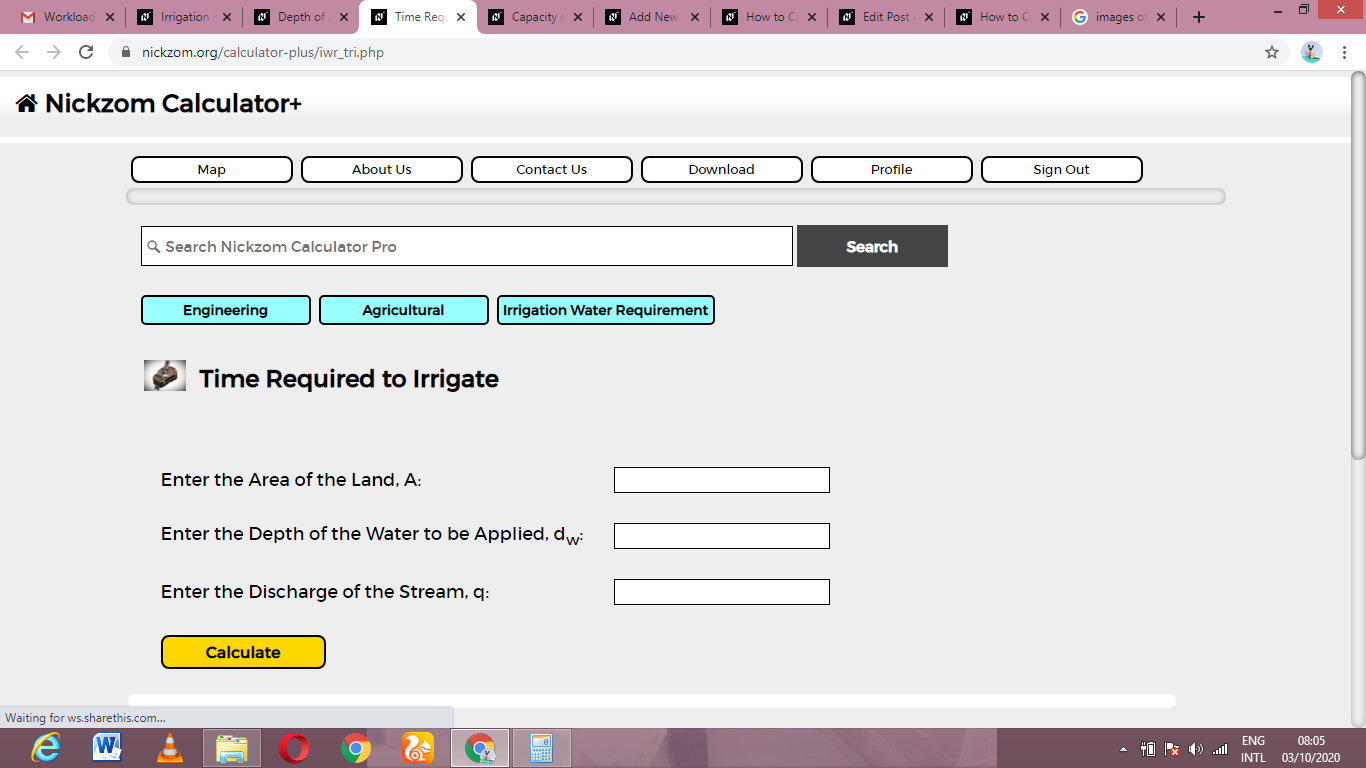Now, enter the values appropriately and accordingly for the parameters as required by the Area of the Land (A) is 14, Depth of the Water to be Applied (dwis 20 and Discharge of the Stream (q) is 10.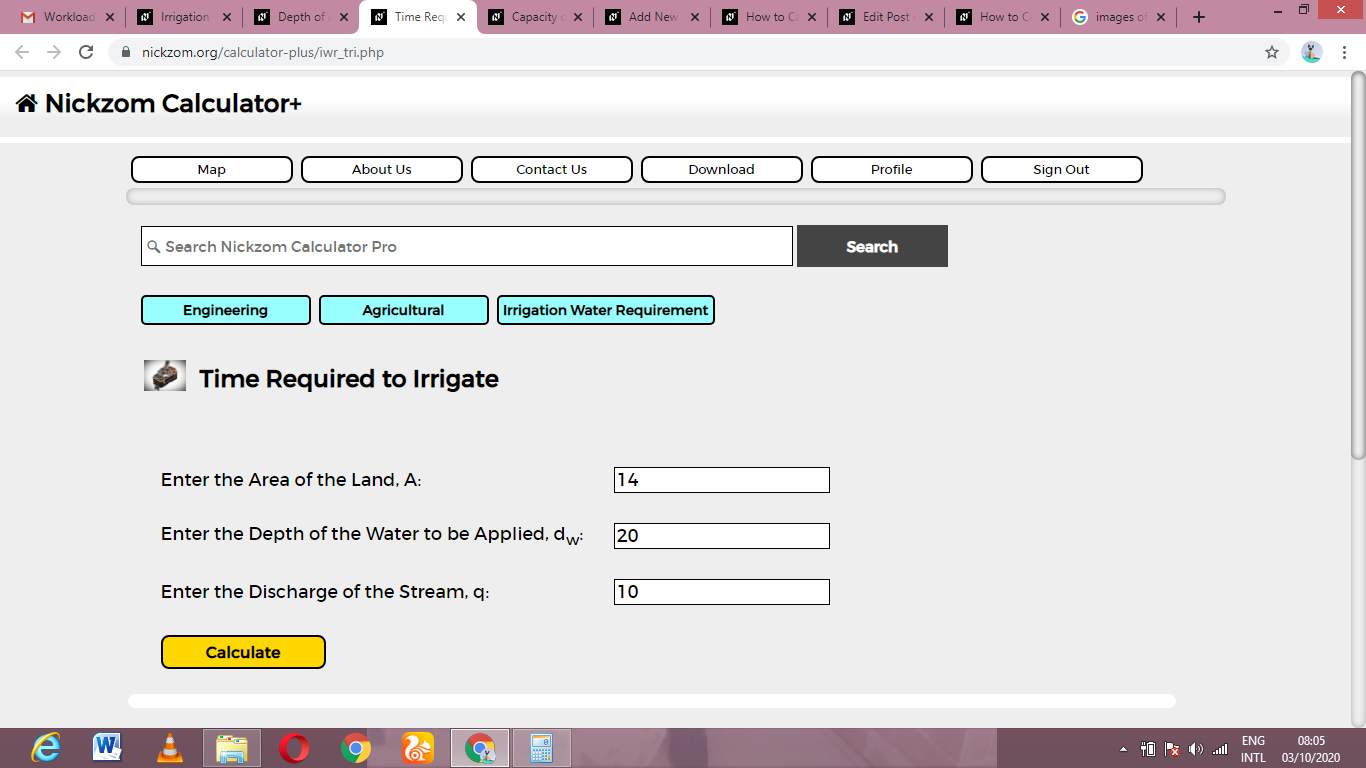Finally, Click on Calculate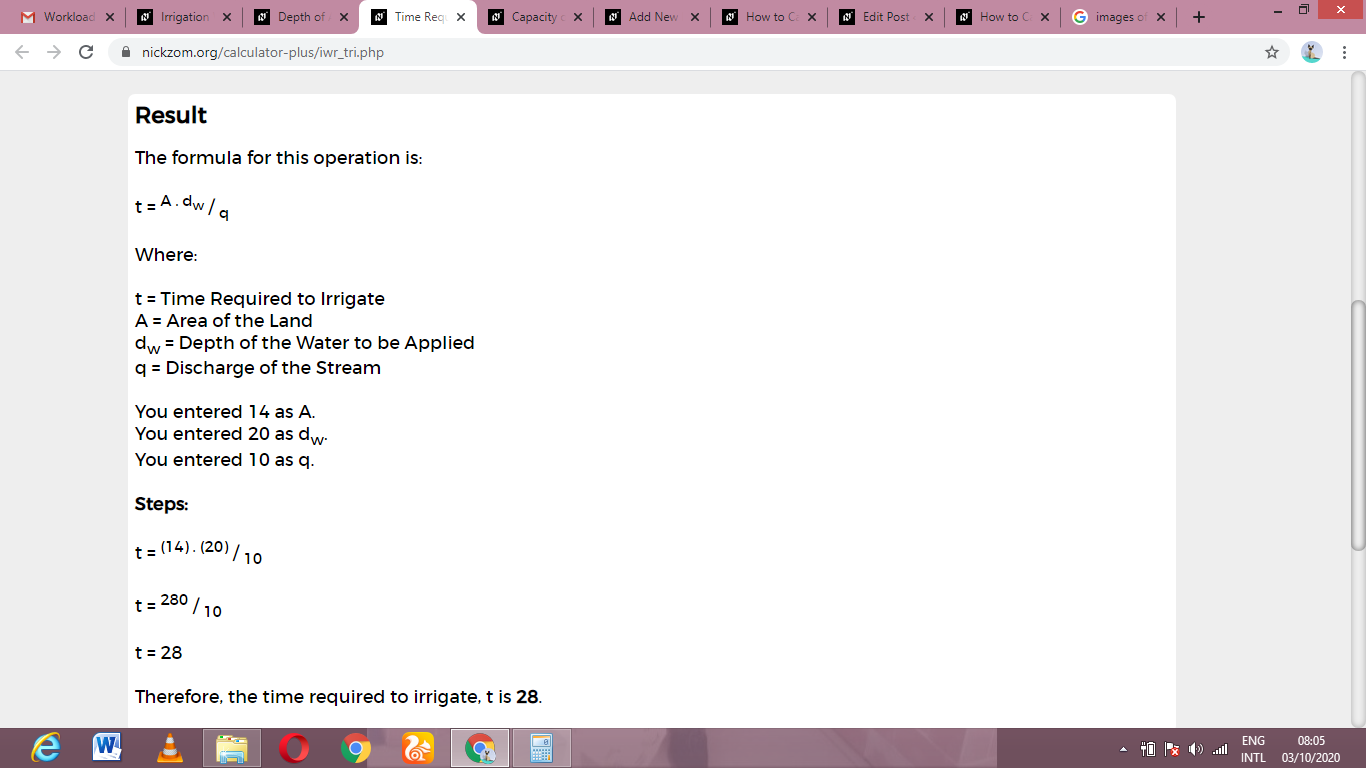As you can see from the screenshot above, Nickzom Calculator– The Calculator Encyclopedia solves for the time required to irrigate and presents the formula, workings and steps too.